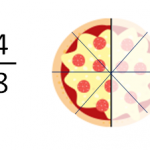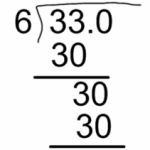Smartick is an advanced online program that teaches kids math and coding in only 15 min. a day

Aug04We will learn about the addition properties through an everyday situation: Preparing a backpack for school. Shall we begin? We look for an empty backpack and open it. First, we put in 3 large notebooks and then 6 small notebooks. How many notebooks are there in the bag? To answer this question, simply add the […]

Aug03

## The Distributive Property of Multiplication

Hello! We’re back again. Today we will look at one of the properties of multiplication: the distributive property of multiplication. As you may know, multiplication has different properties, let’s review them: Commutative property Associative property Identity Property Distributive property Let’s focus on the distributive property of multiplication The distributive property of multiplication states that when […]

Aug01

## “Smartick Is Fun and Flexible”

We care about our students’ opinions and their experiences with Smartick. Today, we’re introducing you to Elise, mother of a Smartick student (also named Elise). How did you find out about Smartick? Through a press article. I had already removed my daughter from paper-based programme, because it was a horrible struggle. I made the decision […]

Jul29

## Why Jeremy Irons Loves Math

Jeremy Irons, at the age of 67, has fallen in love with math thanks to his latest role as the brilliant Cambridge mathematician G. H. Hardy in the recently released film “The Man Who Knew Infinity.” In interviews, Irons has spoken about the seemingly paradoxical connection between emotion and pure thought, and abstract thought and […]

Jul28

## Equivalent FractionsWhat are equivalent fractions? In this post, we are going to understand what equivalent fractions are and how you can find out what fractions are equivalent. Equivalent fractions are fractions that represent the same quantity. For example, which of the following do you believe will be the biggest? What have you figured out? We are […]

Jul27

## Mental Math: Multiplying by 11

In this post, we are going to learn a mental math strategy for multiplying by 11 using a simple method. Mental Math Strategy 1: Multiplying a 2-digit number by 11 It’s only a matter of adding the number’s two digits and then putting the result in between the two numbers. Let’s take a look at some […]

Jul22

## Finding the Average and the Median

In today’s post, we’re going to learn two basic concepts of statistics: average and median. Imagine if we asked all of our classmates what grade their math grade was for this marking period. Assuming that there are 25 people in our class, we’ll collect 25 numbers: It’s a little complicated to process the numbers if […]

Jul21

## Divisibility Criteria for 3, 4, 9 and 11In this post we are going to learn about the criteria of divisibility by 3, 4, 9 and 11. Criteria of divisibility by 3 A number is divisible by 3 when the sum of its digits is a multiple of 3. For example: Is 1098 divisible by 3? We add all the digits of 1098: 1 […]